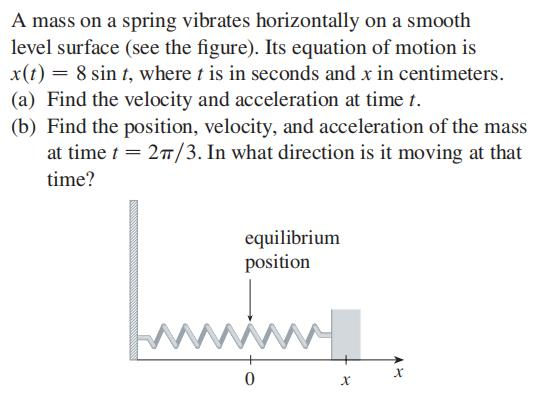### ¿Todavía tienes preguntas de matemáticas?

Pregunte a nuestros tutores expertos
Algebra
PreguntaA mass on a spring vibrates horizontally on a smooth level surface (see the figure). Its equation of motion is $$x ( t ) = 8 \sin t$$ , where $$t$$ is in seconds and $$x$$ in centimeters.

(a) Find the velocity and acceleration at time $$t$$ .

(b) Find the position, velocity, and acceleration of the mass at time $$t = 2 \pi / 3$$ . In what direction is it moving at that time?

$$\left. \begin{array} { l } { \text { (a) } v ( t ) = 8 \cos t , a ( t ) = - 8 \sin t } \\ { \text { (b) } 4 \sqrt { 3 } , - 4 , - 4 \sqrt { 3 } ; \text { to the left } } \end{array} \right.$$